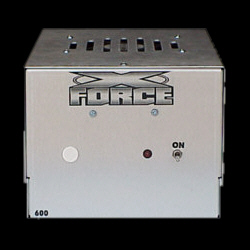Main Shop   |  Wizard Built Products....   |  We will no longer sell Xforce   |  Accessories   |  Used Equipment   |  Meters   |  Wire & Coax   |  Mobiles For sales   |  RF AMP PARTS   |  CALL FOR MORE INFO 773-431-4444   |  customer pics   |# 600-HD *** Amps now have a shorter design to fit nicely under seats***

```Transistors 6 x 2SC2879 Dimensions H **4 1/4**" x L 11 x W 6 3/4" Amp Draw (continuous) 65 Amp Draw (maximum) 120 Class C No SSB Delay No Pre-amp Driver Required X-40 or X-80 (optional) Power Output (RMS) 400 Watts Power Output (PEP) 750 Watts Driven Power (RMS) 600 Watts Driven Power (PEP) 1200 Watts

function AutoUpdatePricePage(e, t, o) {
if(e.elements.hiddenAutoUpdatePrice == undefined) return;
var l = new Number,
t = t ? t : "\$",
n = e.elements.hiddenAutoUpdatePrice.value,
a = e.elements.hiddenProdIdAllotted2Elements.value;
if (a = "spanPriceHolder" + a, n) {
var r = !1;
for (i = 0; i < e.elements.length; i++)
if ("select-one" == e.elements[i].type) {
var d = "div_" + e.name + "_" + e.elements[i].name;
var s = document.getElementById(d).innerText || document.getElementById(d).textContent;
if (void 0 != s)
for (var m = s.split(","), u = m.length, c = 0; u > c; c++) {
var p = m[c].split(":");
if (p == e.elements[i].options[e.elements[i].selectedIndex].value) {
if ("=" == p) l = parseFloat(p), r = !0;
else {
p;
"-" == p ? l = parseFloat(n) - parseFloat(p) : "+" == p ? l = parseFloat(n) + parseFloat(p) : "x" == p && (l = parseFloat(n) * parseFloat(p)), r = !0
}
l = l.toFixed(2), "undefined" != typeof document.getElementById(a).innerText ? document.getElementById(a).innerText = t + l : document.getElementById(a).textContent = t + l, n = l
}
}
}
r || ("undefined" != typeof document.getElementById(a).innerText ? document.getElementById(a).innerText = t + n : document.getElementById(a).textContent = t + n)
}
}
function ChangePriceAndThumb(thisVar,currency_name,regular_price, product_levels){
AutoUpdatePrice(thisVar.form,currency_name,regular_price);
var value = jQuery("#"+ thisVar.id + " option").index(thisVar.options[thisVar.selectedIndex]);
if(product_levels == 1){
jQuery.ajax({
type: "POST",
url: "product_option_image.php",
data: { id: thisVar.options[thisVar.selectedIndex].value }
})
.done(function(thumbimage){
if(thumbimage != 'none')
clsThumbsAutoImageDisplay('0', '67', thumbimage);
});
}
}

Pre Amp

None                         Pre Amp (+\$35.00)

AutoUpdatePricePage(document.prodform67,'','0');

function AutoUpdatePricePage(e, t, o) {
if(e.elements.hiddenAutoUpdatePrice == undefined) return;
var l = new Number,
t = t ? t : "\$",
n = e.elements.hiddenAutoUpdatePrice.value,
a = e.elements.hiddenProdIdAllotted2Elements.value;
if (a = "spanPriceHolder" + a, n) {
var r = !1;
for (i = 0; i < e.elements.length; i++)
if ("select-one" == e.elements[i].type) {
var d = "div_" + e.name + "_" + e.elements[i].name;
var s = document.getElementById(d).innerText || document.getElementById(d).textContent;
if (void 0 != s)
for (var m = s.split(","), u = m.length, c = 0; u > c; c++) {
var p = m[c].split(":");
if (p == e.elements[i].options[e.elements[i].selectedIndex].value) {
if ("=" == p) l = parseFloat(p), r = !0;
else {
p;
"-" == p ? l = parseFloat(n) - parseFloat(p) : "+" == p ? l = parseFloat(n) + parseFloat(p) : "x" == p && (l = parseFloat(n) * parseFloat(p)), r = !0
}
l = l.toFixed(2), "undefined" != typeof document.getElementById(a).innerText ? document.getElementById(a).innerText = t + l : document.getElementById(a).textContent = t + l, n = l
}
}
}
r || ("undefined" != typeof document.getElementById(a).innerText ? document.getElementById(a).innerText = t + n : document.getElementById(a).textContent = t + n)
}
}
function ChangePriceAndThumb(thisVar,currency_name,regular_price, product_levels){
AutoUpdatePrice(thisVar.form,currency_name,regular_price);
var value = jQuery("#"+ thisVar.id + " option").index(thisVar.options[thisVar.selectedIndex]);
if(product_levels == 1){
jQuery.ajax({
type: "POST",
url: "product_option_image.php",
data: { id: thisVar.options[thisVar.selectedIndex].value }
})
.done(function(thumbimage){
if(thumbimage != 'none')
clsThumbsAutoImageDisplay('0', '67', thumbimage);
});
}
}

Ssb & Biasing

None                         SSb & Biasing (+\$75.00)

AutoUpdatePricePage(document.prodform67,'','0');

function AutoUpdatePricePage(e, t, o) {
if(e.elements.hiddenAutoUpdatePrice == undefined) return;
var l = new Number,
t = t ? t : "\$",
n = e.elements.hiddenAutoUpdatePrice.value,
a = e.elements.hiddenProdIdAllotted2Elements.value;
if (a = "spanPriceHolder" + a, n) {
var r = !1;
for (i = 0; i < e.elements.length; i++)
if ("select-one" == e.elements[i].type) {
var d = "div_" + e.name + "_" + e.elements[i].name;
var s = document.getElementById(d).innerText || document.getElementById(d).textContent;
if (void 0 != s)
for (var m = s.split(","), u = m.length, c = 0; u > c; c++) {
var p = m[c].split(":");
if (p == e.elements[i].options[e.elements[i].selectedIndex].value) {
if ("=" == p) l = parseFloat(p), r = !0;
else {
p;
"-" == p ? l = parseFloat(n) - parseFloat(p) : "+" == p ? l = parseFloat(n) + parseFloat(p) : "x" == p && (l = parseFloat(n) * parseFloat(p)), r = !0
}
l = l.toFixed(2), "undefined" != typeof document.getElementById(a).innerText ? document.getElementById(a).innerText = t + l : document.getElementById(a).textContent = t + l, n = l
}
}
}
r || ("undefined" != typeof document.getElementById(a).innerText ? document.getElementById(a).innerText = t + n : document.getElementById(a).textContent = t + n)
}
}
function ChangePriceAndThumb(thisVar,currency_name,regular_price, product_levels){
AutoUpdatePrice(thisVar.form,currency_name,regular_price);
var value = jQuery("#"+ thisVar.id + " option").index(thisVar.options[thisVar.selectedIndex]);
if(product_levels == 1){
jQuery.ajax({
type: "POST",
url: "product_option_image.php",
data: { id: thisVar.options[thisVar.selectedIndex].value }
})
.done(function(thumbimage){
if(thumbimage != 'none')
clsThumbsAutoImageDisplay('0', '67', thumbimage);
});
}
}

Remote

None                         Remote (+\$34.99)

AutoUpdatePricePage(document.prodform67,'','0');

```

For educational purposes only
Phone: 773-431-4444

tony@wizardbuilt.com